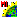# WritePolygonVertices.sml

###Download

#### Syntax Highlighing:

```comments, key words, predefined symbols, class members & methods, functions & classes
```
```# 29 Aug 2012 Cindy Robbins
# For every polygon in your input vector the script gets each line and prints the coordinates of each vertex, line distance and angle.
# Order of vertices listed are based on the line direction. Imagine drawing two identical squares in two different ways. 1) without lifting the pen and 2) draw one side, lift pen, start second line at start of 1st line, etc. ... The two squares will look the same but have different line directions.
#  The 3squares vector in the SimplePolygons.rvc uses a line cartoscript to draw lines - the arrows indicating line direction
clear();
print("Polygon Number, Line Number, X1, Y1,X2, Y2, Distance, Azimuth");
array numeric xArray, yArray, zArray; # will be resized automatically
array numeric linelist;
numeric nPolys, nLines, nVertices;
numeric tempDist, azimuth, elevation;
numeric p,l,v;
numeric obj_x;
numeric obj_y;
numeric map_x;
numeric map_y;
vector Vect;
GetInputVector(Vect);
class Georef georefVect;
georefVect = GetLastUsedGeorefObject(Vect);
nPolys = NumVectorPolys(Vect);
for p = 1 to nPolys {
nLines = GetVectorPolyLineList(Vect, linelist, p);
for l=1 to nLines {
nVertices = GetVectorLinePointList(Vect, xArray, yArray,linelist[l], zArray);
# Georeference for Vect
for v = 1 to nVertices {
obj_x = xArray[v];
obj_y = yArray[v];
ObjectToMap(Vect,obj_x,obj_y,georefVect,map_x,map_y);
xArray[v] = map_x;
yArray[v] = map_y;
}
for v = 1 to nVertices {
if (v <> nVertices) {
Displacement3Dd(xArray[v], yArray[v], 0, xArray[v+1], yArray[v+1], 0, tempDist, azimuth, elevation);
print(p,",", linelist[l],",", xArray[v],",", yArray[v],",", xArray[v+1],",", yArray[v+1],",", tempDist,",", azimuth);
}
}
}
}
CloseVector(Vect);```